# Trigonometry Short Tricks Formula, Trigonometry Hacks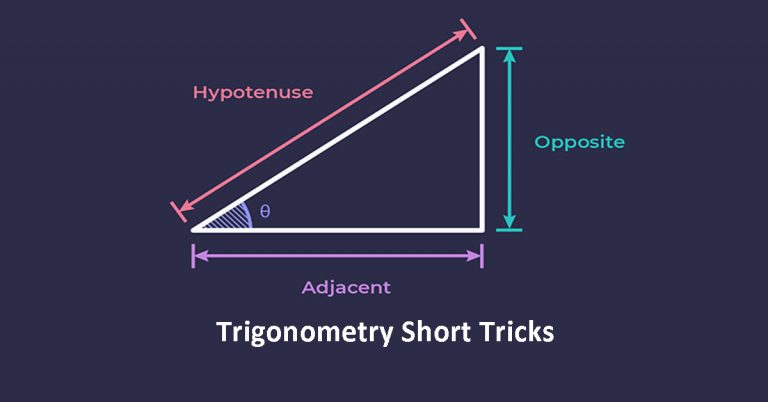In this post, we are going to discuss with you Trigonometry Short Tricks for various competitive exams like SSC, IBPS, CAT, NDA etc. You can solve most questions of trigonometry without using any sin, cos or tan. You can solve the question within 1-2 minutes. The questions that you can solve using this trigonometry hack are related to height and distance only.

## Trigonometry Short Tricks

You just need to understand that in 99% questions related to height and distance in trigonometry, θ (Theta) is either 30°, 45° or 60°. So, basically you have two types of triangles:

### Type I Triangle

In type I triangle, the angle between base and hypotenuse is either 30° or 60° as shown below:You just need to remember that side opposite to 30° angle is x, side opposite to 90° angle is 2x and side opposite to 60° angle is √3x as shown below: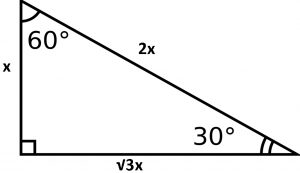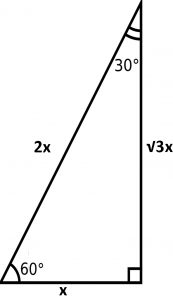Lets’ solve some questions using this trick.

Question: A tree trunk of height 12 meters is erected with the support of a metal chain. An angle of 60° is made by metal chain with the tree trunk. Find the length of the metal chain.

Answer: As per data given in the question, we can draw the picture as shown below: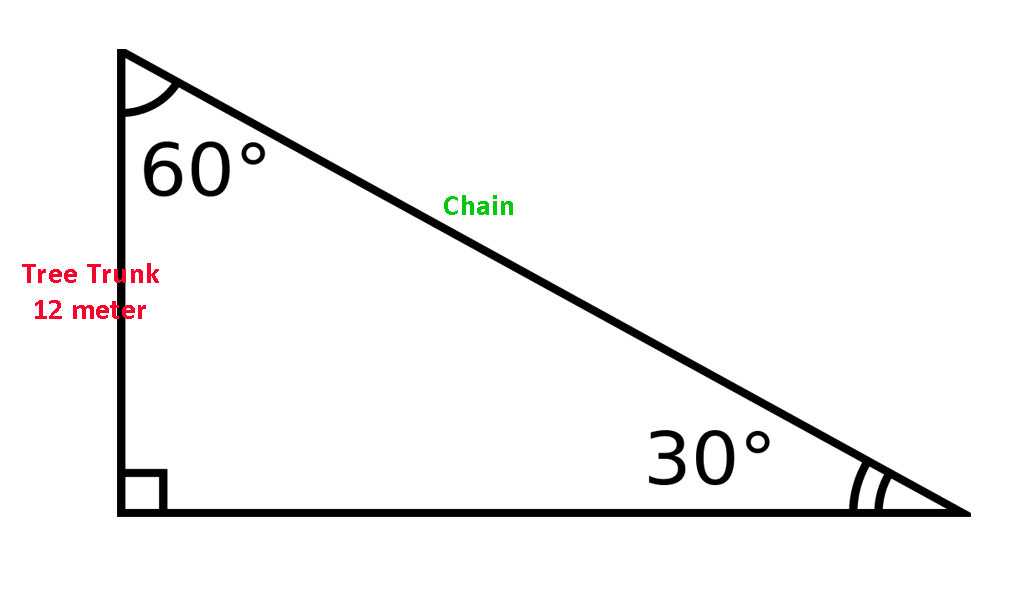As we know from the trick, if side opposite to 30° angle is x, side opposite to 90° angle is 2x. Therefore, length of chain is 2 x 12 = 24 meters.

### Type II Triangle

In type II triangle, the angle between base and hypotenuse is 45° as shown below: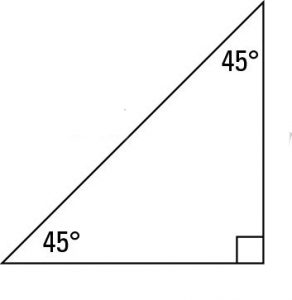You just need to remember that side opposite to 45° angle is x, side opposite to other 45° angle is also x and side opposite to 90° angle is √2x as shown below:Lets’ solve some questions using this trick.

Question: A pole stands vertically on the floor. Point ‘P’ is 120 meters away from the foot of the pole and the angle of elevation is 45° then find the height of the pole.

Answer: As per data given in the question, we can draw the picture as shown below:As we know, sides opposite to 45° angle have same length, therefore height of pole is also 120 meters. See how easy to solve the questions using these trigonometry short tricks.

Check out CAT Course 2022